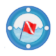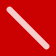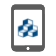920-436-8299 info@dimins.com

# Visualization Library#### DivePort#### ProDiver#### DiveTab## DivePort (61): Charts + Maps + Indicators

### Areas (6)#### Documentation

An Area Chart draws straight lines between data points.

A Multitab Area Chart shows an aggregate of values.

A Multitab Area Chart shows an aggregate of values.

A Multitab Area Chart shows an aggregate of values.

A Spline Area Chart draws curved lines between data points.

A Stacked Area Chart show stacks areas on top of each other, visualizing change in composition or sum total.

### Bars (11)#### Documentation

A Bar Chart displays data as horizontal bars.

A Column Chart displays data as vertical bars.

A Histogram Chart displays the distribution of numeric data across several dimension values.

A MultiTab Bar Chart displays data as horizontal bars.

A MultiTab Column Chart displays data as vertical bars.

A MultiTab Stacked Bar Chart show stacks horizontal bars on top of each other, visualizing change in composition or sum total.

A MultiTab Stacked Bar Chart show stacks vertical bars on top of each other, visualizing change in composition or sum total.

A Sparkcolumn Chart is a simplified column chart without labels or axes. It is ideal for small multiples or finding trends at a glance.

A Stacked Bar Chart or Stacked Column Chart stacks values together, visualizing composition and sum total.

A Stacked Bar Chart or Stacked Column Chart stacks values together, visualizing composition and sum total.

A Waterfall Chart displays rectangles that represent the change in a single value, rather than the magnitude of the value.

### Calendars (1)#### Documentation

A Calendar Heatmatrix displays a single dimension whose daily values display as colors on a calendar.

### Combination (2)#### Documentation

A Combination Chart displays multiple values as superimposed Area, Column, Line, Spline, or Spline Area charts.

A Trellis Chart displays a CrossPlot, where one chart is split into subcharts based on a second dimension called a category. Several subchart types are available, such as bar, column, line, and area.

### Connection (2)#### Documentation

An Arc Chart displays nodes on an axis and arcs connecting the nodes:

The height of an arc is proportional to the distance between its source and target nodes.

The color of an arc indicates the direction of the connection; that is, which connected node is the source and which is the target.

The thickness of an arc line is indicates represents the magnitude of a single value for that connection.

A Chord Chart displays segments around a circle, one for each dimension value, and chords connecting the segments. The thickness of a chord at each end represents the magnitude of a single value where the attached segment is the target. The color of a chord corresponds to the connected segment with the greater output, that is, the greater value where that segment is the source.

### Finance (2)#### Documentation

A Candlestick Chart displays boxes with edges at the open and close values and lines with endpoints at the high and low values. The color of a box represents the direction of movement from open to close: up (white) or down (black).

An Open-High-Low-Close Chart displays vertical lines with endpoints at the high and low values. To the left of each vertical line is a tick mark representing the open value, and to the right another tick mark representing the close value. The color of each line represents the direction of movement from open to close: up (green) or down (red).

### Lines (7)#### Documentation

A Line Chart draws straight lines between data points.

A Line Chart draws straight lines between data points.

A Sparkline Chart is a simplified line chart without labels or axes. They are ideal for small multiples or finding trends at a glance.

A Spline Chart draws curved lines between data points.

A Scatter Plot displays the relationship between two values. Scatter plots are best for visualizing correlation between variables, the clustering or distribution of data, or anomalies and outliers.

A Sparkline Chart is a simplified line chart without labels or axes. They are ideal for small multiples or finding trends at a glance.

A Spline Chart draws curved lines between data points.

#### Stringline Chart

A Stringline Chart is a variant of a MultiTab Line Chart that displays multiple values sliced by a second dimension, called a category. Commonly used to visualize timing of public transit routes, stringline charts are best for showing groups of processes that progress through a series of checkpoints.

### MultiTab (2)#### Documentation

A Heatmatrix uses a color scale to represent a single value for each cell of a matrix.

A Mosaic Chart displays tiles in a matrix, where the size of each tile is determined by the magnitude of a single value.

### Pies (2)#### Documentation

A donut chart displays values of a single dimension as wedges. By default, they display up to 12 values. If there are more than 12 values, the chart displays the first 11 values, and combines the remaining values in a 12th wedge called Other. They are best for visualizing percent composition or part-to-whole relationships.

A pie chart displays values of a single dimension as wedges. By default, they display up to 12 values. If there are more than 12 values, the chart displays the first 11 values, and combines the remaining values in a 12th wedge called Other. They are best for visualizing percent composition or part-to-whole relationships.

### Statistics (2)#### Documentation

A Box Plot or a Horizontal Box Plot displays the minimum, maximum, median, and first and third quartiles of a distribution for each dimension value. It can also show outliers.

A Box Plot or a Horizontal Box Plot displays the minimum, maximum, median, and first and third quartiles of a distribution for each dimension value. It can also show outliers.

### Maps (4)#### Documentation

Boundary maps use country, province, state, county, or postal code data. Predefined regions of the map are color-coded according to the data summarized over a region.

Line maps use longitude and latitude coordinates to show origin-destination data by drawing lines on a map.

Point maps use longitude and latitude coordinates to show data points. Summarized data concentration is shown using color or sizing of the data points.

Heat maps use longitude and latitude coordinates to show data concentration using colors.

### Indicators (20)#### Documentation

A horizontal bar chart containing threshold and target values. Use bullets to display summaries that have multiple meaningful values. For example, trends over time, relative standing within a group, or a metric with multiple targets.

A horizontal bar chart that displays values to the left (below) and to the right (above) of a designated baseline. Use plus-minus indicators to display values with a single baseline where the amount by which you exceed or fail to meet the value is important.

A vertical bar chart containing threshold and target values. Use bullets to display summaries that have multiple meaningful values. For example, trends over time, relative standing within a group, or a metric with multiple targets.

A vertical bar chart that displays values to the above or below a designated baseline.

A circular numeric scale that indicates values in terms of percentage. Use a circular gauge with single unit data to indicate progress or activity.

An arc that indicates values in terms of percentage. Use a fill gauge with single unit data to indicate progress or activity.

A 180° arc with colored performance thresholds and a needle or arrow to indicate in where in the scale the value falls. Use gauges when three ranges are needed. For example, unacceptable, acceptable, and exceptional, and you want to use a scale other than the threshold values.

A 90° arc with colored performance thresholds and an arrow to indicate where in the scale the value falls.

A 90° arc with colored performance thresholds and an arrow to indicate where in the scale the value falls.

A 240° arc with colored performance thresholds and a needle to indicate where in the scale the value falls.

A designated set of images you can use to display up to four thresholds. Link each range to a different image. You can upload custom image sets.

A set of two to five colored circles indicating where a value falls within a set of threshold ranges. Use racetrack indicators to provide context to the values displayed.

A set of two to five lights indicating where a value falls within a set of threshold ranges. Use racetracks to provide context to the data displayed.

A single shape that changes color based on whether the value is above or below specified thresholds. You can choose a circle, square, triangle, or arrow shape for the alert. Use alerts to provide context to data on a tabular display or as free standing portlets.

A single rectangular shape with customizable visual elements that change based on various thresholds. Use rectangles when you want to display thresholds for different summary values at the same time.

A right-pointing arrow that changes direction based on changes over time, with no change pointing right and all other values pointing up or down at an angle (depending on whether the change in value is positive or negative). Use the slope when the direction of change over time is the only element that matters.

A box containing the data value is displayed on a left-to-right track. The position of the data box is relative to the numbers at each end of the track. Use the slider when you want to indicate the value position in a range to provide context to a number of values.

A thick line with an arrow that shows the data value relative to thresholds.

A box containing the data value is displayed on a vertical track. The position of the data box is relative to the numbers at each end of the track. Use the slider when you want to indicate the value position in a range to provide context to a number of values.

An alphanumeric string that is colored to indicate its value’s relationship to the thresholds. Use the text indicator to indicate a value’s acceptability without added graphics.

NOTE: No data and null values appear as “- -”## ProDiver (52): Charts + Spider + Indicators

### Areas (2)#### Documentation

Basic plot graphs display data for a single dimension and up to 16 summaries. They consist of a series of plotted points that correspond to summary values being displayed.

Basic plot graphs display data for a single dimension and up to 16 summaries. They consist of a series of plotted points that correspond to summary values being displayed.

### Bars (12)#### Documentation

A bar plot graph displays data as horizontal bars.

A bar plot graph displays data as horizontal 3-D bars.

Cross plot graphs shows data that pertains to two dimensions simultaneously.

Cross plot graphs shows data that pertains to two dimensions simultaneously.

Cross plot graphs shows data that pertains to two dimensions simultaneously.

Cross plot graphs shows data that pertains to two dimensions simultaneously.

Stack plot graphs display summary values in a stacked format.

Stack plot graphs display summary values in a stacked format.

Stack plot graphs display summary values in a stacked format.

Stack plot graphs display summary values in a stacked format.

Stack plot graphs display summary values in a stacked format.

Stack plot graphs display summary values in a stacked format.

### Bubbles (2)#### Documentation

Cross plot graphs shows data that pertains to two dimensions simultaneously.

Cross plot graphs shows data that pertains to two dimensions simultaneously.

### Calendars (3)#### Documentation

Calendar View with Bar Plots—Shows a bar (or point) representing the relative size of summary data on each day of the calendar.

Calendar Active View—Shows whether or not summary data is available for each day

Shows color-coded data that indicates a range of values

### Control (7)#### Documentation

Control chart graphs show statistical background data for a single dimension. They can be used to observe trends.

Control chart graphs show statistical background data for a single dimension. They can be used to observe trends.

Control chart graphs show statistical background data for a single dimension. They can be used to observe trends.

Control chart graphs show statistical background data for a single dimension. They can be used to observe trends.

Control chart graphs show statistical background data for a single dimension. They can be used to observe trends.

Control chart graphs show statistical background data for a single dimension. They can be used to observe trends.

Control chart graphs show statistical background data for a single dimension. They can be used to observe trends.

### Indicators (4)#### Documentation

A 180° arc with colored performance thresholds and a needle or arrow to indicate in where in the scale the value falls. Use gauges when three ranges are needed. For example, unacceptable, acceptable, and exceptional, and you want to use a scale other than the threshold values.

A 240° arc with colored performance thresholds and a needle to indicate where in the scale the value falls.

A designated set of images you can use to display up to four thresholds. Link each range to a different image.

A thermometer with colored performance thresholds.

### Lines (7)#### Documentation

A line plot graph draws straight lines between data points.

A spline plot graph draws curved lines between data points.

A spline plot graph draws 3-D curved lines between data points.

Cross plot graphs shows data that pertains to two dimensions simultaneously.

Cross plot graphs shows data that pertains to two dimensions simultaneously.

Cross plot graphs shows data that pertains to two dimensions simultaneously.

Cross plot graphs shows data that pertains to two dimensions simultaneously.

### Pies (4)#### Documentation

Pie plot graphs display up to 16 values for a single dimension. If there are more than 16 values, the graph displays the first 15 values listed in the tabular display, and combines the remaining values in a 16th wedge called Other.

Pie plot graphs display up to 16 values for a single dimension. If there are more than 16 values, the graph displays the first 15 values listed in the tabular display, and combines the remaining values in a 16th wedge called Other.

Doughnut plot graphs display up to 16 values for a single dimension. If there are more than 16 values, the graph displays the first 15 values listed in the tabular display, and combines the remaining values in a 16th wedge called Other.

Doughnut plot graphs display up to 16 values for a single dimension. If there are more than 16 values, the graph displays the first 15 values listed in the tabular display, and combines the remaining values in a 16th wedge called Other.

### Points (3)#### Documentation

Basic plot graphs display data for a single dimension and up to 16 summaries. They consist of a series of plotted points that correspond to summary values being displayed.

Cross plot graphs shows data that pertains to two dimensions simultaneously.

Cross plot graphs shows data that pertains to two dimensions simultaneously.#### Documentation

Radar graphs display summary values as radius points from a common central axis.

Radar graphs display summary values as radius points from a common central axis.

Radar graphs display summary values as radius points from a common central axis.

### Scatter (3)#### Documentation

Scatter graphs display data for two summaries across a single dimension.

Scatter graphs display data for two summaries across a single dimension.

Scatter graphs display data for two summaries across a single dimension.

### Spider (1)#### Documentation

A Closed spider graph is a chart that consists of a sequence of evenly-spaced spokes, with each spoke representing a variable (row). They can be used to display unrelated data because the data points are relative to the column threshold and goal values as opposed to each other. A line is drawn connecting the data values for each spoke. This gives the plot a spider web-like appearance.

### Time (1)#### Documentation

Time Graphs show linear bars that represent a range of time.## DiveTab (8): Charts + Indicators

### Bars (2)#### Documentation

A Bar Chart uses vertical bars to represent numeric quantities of data.

An H Bar Chart, or Horizontal Bar Chart, uses horizontal bars to present numeric quantities of data.

### Lines (1)#### Documentation

A Line Chart uses points on a graph and a line between them to show the relationship between numerical data values.

### Pies (1)#### Documentation

A Pie Chart uses percentages of a circle to show the relationship that numerical data values have to the total.

### Indicators (4)#### DiveTab

An alert identifies whether a value is above, below, or between certain thresholds. Alerts use symbols and colors to indicate the values that exceed these thresholds, so you can quickly see the outliers.

#### Documentation

A bullet column displays horizontal bars that demonstrate the numerical values for a specific summary. With bullet columns, DiveTab users can view the information graphically. By providing visual information, it is easier to analyze the numerical data by comparing it to the other values shown.

#### Documentation

A designated set of images you can use to display up to four thresholds. Link each range to a different image.

#### Documentation

An alphanumeric string that is colored to indicate its value’s relationship to the thresholds. Use the text indicator to indicate a value’s acceptability without added graphics.